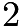# October 2018 Brain Puzzler Solution

Q: A Math quiz has$3$ multiple-choice questions, each with$3$ choices. A student passes the quiz with$2$ or more correct questions. If the student randomly picks their answers, what is the probability of passing the quiz?

A: 7/27  = 1/27 + 6/27
Scenario 1: The student gets all 3 correct (1/3) x (1/3) x (1/3) = 1/27
Scenario 2: The student gets 2 correct.  1/3 x 1/3 x 2/3 but this can happen 3 different ways as the student could get the 1st one incorrect or the 2nd or the 3rd.
so (1/3)x (1/3) x (2/3) x 3 = 6/27
1/27 + 6/27 = 7/27.